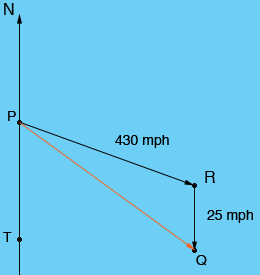Name: Jimmie Who is asking: Student Level of the question: Secondary Question: An aircraft going from city A to city B on a bearing of S69E (degrees) is traveling at a speed of 430 mph. The wind is blowing out of the north to south at a speed of 25 mph. Find the ground speed and the planes true heading. Hi Jimmie, In the diagram below the measure of the angle RPT is 69o , the vector PR represents the speed and air direction of the plane and the vector RQ represents the wind. Thus the length of the vector PQ represents the ground speed of the plane and the angle QPT gives the true heading.Since PT and RQ are parallel the measure of the angle QRP is 180o - 69o = 111o. Do you know a trigonometric expression that will allow you to find the length of PQ? Penny